Test: Mutual Inductance - 2

# Test: Mutual Inductance - 2

Test Description

## 10 Questions MCQ Test Electromagnetic Fields Theory (EMFT) | Test: Mutual Inductance - 2

Test: Mutual Inductance - 2 for Electrical Engineering (EE) 2023 is part of Electromagnetic Fields Theory (EMFT) preparation. The Test: Mutual Inductance - 2 questions and answers have been prepared according to the Electrical Engineering (EE) exam syllabus.The Test: Mutual Inductance - 2 MCQs are made for Electrical Engineering (EE) 2023 Exam. Find important definitions, questions, notes, meanings, examples, exercises, MCQs and online tests for Test: Mutual Inductance - 2 below.
Solutions of Test: Mutual Inductance - 2 questions in English are available as part of our Electromagnetic Fields Theory (EMFT) for Electrical Engineering (EE) & Test: Mutual Inductance - 2 solutions in Hindi for Electromagnetic Fields Theory (EMFT) course. Download more important topics, notes, lectures and mock test series for Electrical Engineering (EE) Exam by signing up for free. Attempt Test: Mutual Inductance - 2 | 10 questions in 30 minutes | Mock test for Electrical Engineering (EE) preparation | Free important questions MCQ to study Electromagnetic Fields Theory (EMFT) for Electrical Engineering (EE) Exam | Download free PDF with solutions
 1 Crore+ students have signed up on EduRev. Have you?
Test: Mutual Inductance - 2 - Question 1

### Two coils having self-inductances of 10 mH and 40 mH are mutually coupled. What is the maximum possible mutual inductance?

Detailed Solution for Test: Mutual Inductance - 2 - Question 1

Concept:
The mutual inductance is given by: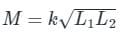where, M = Mutual inductance
L = Self-inductance
k = Coefficient of coupling
The value of k lies between 0 and 1.
The maximum value of mutual inductance is possible for k = 1.
Calculation:
Given, L1 = 10 mH
L2 = 40 mH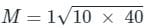M = 20 mH

Test: Mutual Inductance - 2 - Question 2

### The coefficient of coupling between two coils is 0.45. The first coil has an inductance of 75 mH and the second coil has an inductance of 105 mH. What is the mutual inductance between the coils?

Detailed Solution for Test: Mutual Inductance - 2 - Question 2

Concept:
Coefficient of Coupling (k):

The coefficient of coupling (k) between two coils is defined as the fraction of magnetic flux produced by the current in one coil that links the other.
Two coils have self-inductance L1 and L2, then mutual inductance M between them then Coefficient of Coupling (k) is given by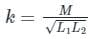Where,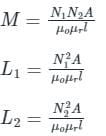N1 and N2 is the number of turns in coil 1 and coil 2 respectively
A is the cross-section area
l is the length
Calculation:
Given,
L1 = 75 mH
L2 = 105 mH
k = 0.45
From the above concept,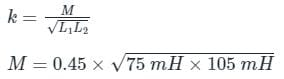M = 39.93 mH

Test: Mutual Inductance - 2 - Question 3

### The self inductance of two coils are 4mH and 9mH respectively. If the coefficient of coupling is 0.5, the mutual inductance between the coils is _____

Detailed Solution for Test: Mutual Inductance - 2 - Question 3

Concept:
Coefficient of Coupling (k):

The coefficient of coupling (k) between two coils is defined as the fraction of magnetic flux produced by the current in one coil that links the other.
Two coils have self-inductance L1 and L2, then mutual inductance M between them then Coefficient of Coupling (k) is given by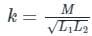Where,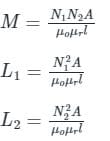N1 and N2 is the number of turns in coil 1 and coil 2 respectively
A is the cross-section area
l is the length
Calculation:
Given,
L1 = 4mH
L2 = 9mH
k = 0.5 H
From the above concept,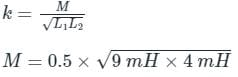M = 3 mH

Test: Mutual Inductance - 2 - Question 4

The total inductance of two coupled coils in the ‘series aiding’ and ‘series opposing’ connections are 1.4 × 10-3 Henry and 0.6 × 10-3 Henry, respectively. The value of mutual inductance will be:

Detailed Solution for Test: Mutual Inductance - 2 - Question 4

Concept:
The equivalent inductance of two inductors in a series connection can be calculated as
For mutual aiding (when polarity dots are present at the same ends)
Leq = L1 + L2 + 2M
For mutual opposing (when polarity dots are present at the opposite ends)
Leq = L1 + L2 − 2M

Calculation:
For series aiding, the equivalent inductance is 1.4 mH, i.e.
1.4 mH = L+ L2 + 2M   ---(1)
For series opposing, the equivalent inductance is 0.6 mH, i.e.
0.6 mH = L1 + L2 − 2M   ---(2)
Subtracting the two equations, we get:
1.4 mH - 0.6 mH = 4 M
4 M = 0.8 mH
M = 0.2 mH = 0.2 × 10-3 Henry

*Answer can only contain numeric values
Test: Mutual Inductance - 2 - Question 5

An air-core radio-frequency transformer as shown has a primary winding and a secondary winding. The mutual inductance M between the windings of the transformer is ______ μH.
(Round off to 2 decimal places.)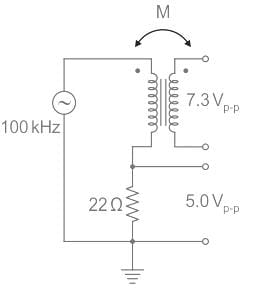Detailed Solution for Test: Mutual Inductance - 2 - Question 5

Concept:
Mutual Inductance:

When two coils are placed close to each other, a change in current in the first coil produces a change in magnetic flux, which cuts not only the coil itself but also the second coil as well. The change in the flux induces a voltage in the second coil, this voltage is called induced voltage and the two coils are said to have a mutual inductance.
Consider a pair of coupled inductors with self-inductance L1 and L2, magnetically coupled through coupling coefficient k.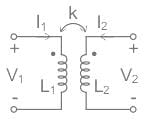Input and output voltage expressions are given as
V1 = jωL1I1 + jωMI2   ...(1)
V2 = jωL2I2 + jωMI1   ...(2)
Where,
ω = 2πf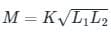M = Mutual inductance
L1 = Inductance of coil one
L2 = Inductance of coil two
Calculation: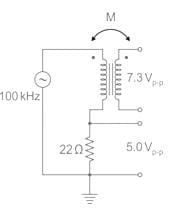In the given circuit secondary is open-circuited, so I2 = 0 A
Given secondary voltage V2 = 7.3 Vp-p
The output voltage expression from equation(2) is given as
V2 = jωMI1  ....(3)
The given voltage across the 22 Ω resistor is 5 Vp-p
So primary current is calculated as I1 = 5 / 22 Ap-p
From equation(3)
|V2| = 2π f M I1
7.3 = 2 × π ×  100 × 103 × M × (5/22)
M = 51.12 μH

Test: Mutual Inductance - 2 - Question 6

Determine the mutual inductance between two coils when a current changing at 20 A/s in one coil induces an EMF of 50 mV in the other.

Detailed Solution for Test: Mutual Inductance - 2 - Question 6

Concept:
The coefficient of mutual induction of two coils is numerically equal to the magnetic flux linked with one coil when unit current flows through the neighboring coil.
If E is the induced emf in the coil at any instant of time, then from the laws of electromagnetic induction: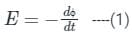ϕ = M × I
M is the coefficient of mutual induction
I is the current.
Equation (1) becomes: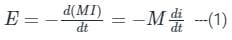Calculation:
Given:
di/dt = 20
E = 50 mV
From equation (1) M can be calculated as:
50 × 10−3 = −M × 20
M = - 2.5 mH
So M = 2.5 mH

Test: Mutual Inductance - 2 - Question 7

Two inductive coils which are close to each other have a mutual inductance of 0.3 H. Current through one coil is increased from 1 A to 4 A in 0.03 s. The voltage induced in the other coil is:

Detailed Solution for Test: Mutual Inductance - 2 - Question 7

Concept:
Let current through the two coils are I1 & I2 and the voltages are V1 & V2 respectively.
∴ The voltage induced in the 2nd coil due to current in the first coil can be calculated as: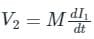Where M = Mutual inductance between two inductive coils
dI1 = change in current
dt = change in time
Calculation:
Mutual inductance (M) = 0.3
Change in current (dI1) = 4 – 1 = 3 A
Change in time (dt) = 0.03 sec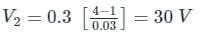Test: Mutual Inductance - 2 - Question 8

Two coupled coils have self inductances L1 = 10 mH and L2 = 20 mH.
The co-efficient of coupling (K) being 0.75 in the air. Voltage in the second coil when the current in circuit is given by I = 2 sin (314t) A is _______

Detailed Solution for Test: Mutual Inductance - 2 - Question 8

MUTUAL INDUCTION:
If two coils of wire are brought into close proximity with each other so that the magnetic field from one links with the other, a voltage will be generated in the second coil as a result.
This is called mutual induction: when voltage impressed upon one coil induces a voltage in another.
Mutual inductance formula-
Vm = M (dI1 / dt)
where Vm = voltage induced in the secondary coil
I1 = is the current flowing in the primary foil
M = mutual inductance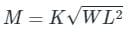where K = coupling coefficient
Given:
L1 = 10 mH
L2 = 20 mH
K = 0.75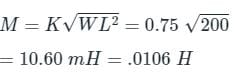Voltage in the second coil: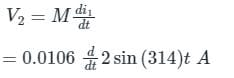= 6.66 cos (314 t) volt

Test: Mutual Inductance - 2 - Question 9

Two coils having self-inductance of L1 and L2, respectively, are magnetically coupled. The maximum possible value of mutual inductance between the coils is

Detailed Solution for Test: Mutual Inductance - 2 - Question 9

Concept:

• The inductor is an electrical component that is capable of storing electrical energy in the form of magnetic energy.
•  The property of an electrical component that causes an emf to be generated by changing the current flow is known as inductance. Inductance is of two types
• Self-inductance: This is the phenomena in which change in electric current produce an electromotive force in the same circuit, and is given by

ϕ = L I
Where ϕ  = Magnetic flux, L = Self inductance, I = Current
Mutual inductance: This is the phenomena in which change in flux linked with one circuit produce an emf in another coil and is given by
ϕ = MI
Where M = mutual inductance, ϕ  = magnetic flux, I = Current
The coupling coefficient is the ratio of mutual inductance to the maximum possible value of mutual inductance and is given by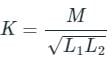Where M = Mutual inductance, L1, L2 = Self-inductance of coil 1 and coil 2 respectively
The maximum possible value of mutual inductance is at K = 1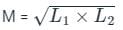Test: Mutual Inductance - 2 - Question 10

Mutual inductance between two closely coupled coils is 2 H. Now, if the number of turns in one coil is reduced by 50 percent and those of the other coil is doubled then, new value of mutual inductance is:

Detailed Solution for Test: Mutual Inductance - 2 - Question 10

Concept:
The inductance of a coil is given by,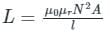μ0 is magnetic permeability of free space
μr is relative permeability
N is number if turns
A is cross-sectional area
l is length
Calculation: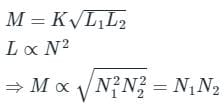Given that M = 2 H
If the turns of one coil are decreased to half and the other is doubled.
Now the new value of the mutual inductance would be 2 H.

## Electromagnetic Fields Theory (EMFT)

11 videos|46 docs|62 tests
 Use Code STAYHOME200 and get INR 200 additional OFF Use Coupon Code
Information about Test: Mutual Inductance - 2 Page
In this test you can find the Exam questions for Test: Mutual Inductance - 2 solved & explained in the simplest way possible. Besides giving Questions and answers for Test: Mutual Inductance - 2, EduRev gives you an ample number of Online tests for practice

## Electromagnetic Fields Theory (EMFT)

11 videos|46 docs|62 tests